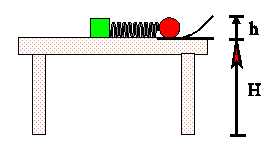Teaching Physics with the Physics Suite Edward F. Redish

Launching a ball with a spring

A child's game consists of a block that attaches to a table with a suction cup, a spring connected to that block, a ball, and a launching ramp. By compressing the spring, the child can launch the ball up the ramp.

The spring has a spring constant k, the ball has a mass m, and the ramp rises a height h. The spring is compressed a distance s in order to launch the ball. When the ball leaves the launching ramp its velocity makes an angle q with respect to the horizontal.1. Assuming that friction and air resistance can be ignored for the purposes of this problem, describe the changes in the forms of energy in the system from the time the spring is compressed until the ball first hits the ground.
2. Calculate the vector velocity of the ball when it leaves the launching ramp. Be sure to specify your coordinate system.
3. The spring constant = 32 N/m, the spring's compression is 5 cm, the ball's mass is 20 grams, the height of the ramp is 10 cm, and the top of the table is 1 m above the floor. With what speed will the ball hit the floor? (Use gĀ=Ā10 m/s2).

Not finding what you wanted? Check the Site Map for more information.